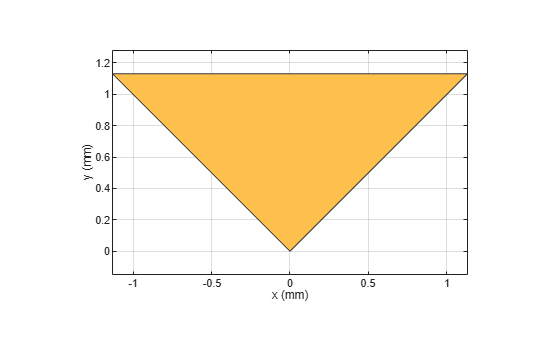# delta

Create delta shape

## Description

Use `delta` object to create a delta shape on the X-Y plane.

## Creation

### Syntax

``deltashape = delta``
``deltashape = delta(Name=Value)``

### Description

example

````deltashape = delta` creates a delta shape on the X-Y plane.```
````deltashape = delta(Name=Value)` sets Properties using one or more name-value arguments. For example, `delta(ReferencePoint=[1 1])` creates a delta shape with the reference point at `[1 1]`. Properties not specified retain their default values.```

## Properties

expand all

Name of the delta shape, specified as a character vector or string scalar.

Example: `deltashape = delta(Name='deltaShape')`

Data Types: `char`

Reference point of delta shape in Cartesian coordinates, specified as a two-element vector of nonnegative elements. Use the reference point to modify the shape relative to its initial position.

Example: `deltashape = delta(ReferencePoint=[1 1])`

Data Types: `double`

Outer radius of the delta, specified as a positive scalar in meters.

Example: `shape = delta(OuterRadius=0.0024)`

Data Types: `double`

Inner radius of the delta, specified as a nonnegative scalar in meters. This value truncates the delta from the tip.

Example: `shape = delta(InnerRadius=0.3)`

Data Types: `double`

Angel of the delta shape, specified as a positive scalar in degrees. The value of the angle must be greater than 0 degrees and lesser than 180 degrees.

Example: `shape = delta(Angle=50)`

Data Types: `double`

## Object Functions

 `add` Boolean unite operation on two RF PCB shapes `and` Shape1 & Shape2 for RF PCB shapes `area` Calculate area of RF PCB shape in square meters `intersect` Boolean intersection operation on two RF PCB shapes `mesh` Change and view mesh properties of metal or dielectric in PCB component `minus` Shape1 - Shape2 for RF PCB shapes `plus` Shape1 + Shape2 for RF PCB shapes `rotate` Rotate RF PCB shape about defined axis `rotateX` Rotate RF PCB shape about x-axis `rotateY` Rotate RF PCB shape about y-axis and angle `rotateZ` Rotate RF PCB shape about z-axis `subtract` Boolean subtraction operation on two RF PCB shapes `scale` Change size of RF PCB shape by fixed amount `show` Display PCB component structure or PCB shape `translate` Move RF PCB shape to new location

## Examples

collapse all

Create a delta shape with default properties.

`deltashape = delta`
```deltashape = delta with properties: Name: 'mydelta' ReferencePoint: [0 0] OuterRadius: 0.0016 InnerRadius: 0 Angle: 90 ```

View the shape.

`show(deltashape)`## Version History

Introduced in R2021b